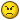# Finding equations from graphs

## Recommended Posts

I'm in the middle of revision for my final GCSE maths exam.

So far its been alright but today I've come across something that is not covered in any of the books I'm using.

I'm assuming its an A* question (since "Introduction to modelling" comes up right at the end of one of the revision textbooks.)

Here's the question -

d = av + bv^2

Find the values of a and b

co-ordinates on the expotential graph -

(40,120) (60,240)

I know the answer is (because they are provided in a separate booklet)

a = 1

b= 1/20

Whats the methodology? I know that for a straight line - finding the gradient would give me the answer. I've tried rearranging the equation.

I'm running out of time fast so I would prefer no 'sidewinding.'

e.g. - If you don't learn yourself . . .blah blah.

I just need the method so I'll be able to tackle the question if it comes up.

Much appreciated

##### Share on other sites

It's pretty simple really you've done Simultaneous equations, you have 2 sets of values for d and v, so plug those in each time and solve for a and b like so -

$120 = 40a + 1600b$ (eq a)

$240 = 60a + 3600b$ (eq b)

$180 = 60a + 2400b$ (eq c = eq b x 1.5 )

So (eq a) - (eq c) -

$60 = 1200b$

${60 \over 1200} = b = {1 \over 20 }$ ( Got b)

$120 = 40a + 1600 \times {1 \over 20}$

$120 = 40a + 80$

$120 - 80 = 40a$

$40 = 40a$

$a = 1$

##### Share on other sites

Why does this keep happening to me !!!It was as easy as that !!!

Thanks anyway Aeternus.

You've knocked some sense into me.

They'res some similar questions involving finding the trig equations to graphs.

I'm sure I'll cope now.

##### Share on other sites

• 2 weeks later...

Sorry - there might be some more maths questions after this (until my exams finish - end of June)

Cloud has two lists of numbers.

The mean of the numbers in the first list is p

The mean of the numbers in the second list is q.

He combines the lists.

He says:

"The mean of the new list of numbers is equal to p+q "

____

2

One of two conditions must be satisfied for him to be correct

Write both conditions.

_______________________________________________________________

Thats the first question.

The 2nd one - (less bullshit)

David collected 1p and 2p coins in a jar.

There are 374 coins in the jar.

The coins have a total value of £5.02.

Use algebra to find how many 1p and 2p coins there are in the jar.

___________________________________________________________

I'm pretty sure I need to construct yet another simultaneous equation for the 2nd one. Its not that I'm lazy. I just have 8 other subjects.

Its pretty stressful right now. Please - someone help.

Much appreciated.

(And I'll know I'll kick myself if someone does reply)

##### Share on other sites

Umm.. Cloud are you doing GCSEs?

Anyway, I think I have the answer for no.2

I'm a not a great mathematician, but I think I've got the methgod right.

Let's say that the number of 1p cons = x

AND

the number of 2p coins = y.

Then,

x + 2y = 502 (1)

x + y = 374 (2)

Eliminate x to obtain -

y = 128.

Substitute y value into equation to obtain x -

x + y =374

x + 128 = 374

x = 374-128

x = 246.

Good luck.

##### Share on other sites

When I tried to work it out I wrote:

x+ 2y = 374

x+y=502

I knew something was wrong.

Thks Vrus.

Could someone do the first one? Its p+q/2 (if that isn't clear).

So far I have come up with - they all have to be positive numbers. But that is wrong because it doesn't really matter + or -.

## Create an account

Register a new account